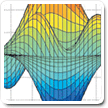Cody

# Problem 892. Solve Rubik's Cube - Up to Two Face Moves

A standard Rubik's Cube is shown in 3-D and also unfolded to identify the specific Tile-face/Vector numbering.

The faces are White-Up / Red-Left / Blue-Front / Orange-Right / Yellow-Down / Green-Back (ULFRDB)

Moves are denoted as F for clockwise rotation of the Front face. F' is CCW and F2 is F twice. The loaded function r_new=rubick_rot(mov,r) implements moves 1-18: UFDLBRU'F'D'L'B'R'U2F2D2L2B2R2.```Input: (rubik)
```
```rubik: row vector of size 54
(The cube started [L=0,U=1,F=2,D=3,B=4,R=5] and then received one or two face moves.
```
```Output: mov (A row vector of one or two of values {1:18})
mov: is a vector values 1:18 representing the moves in order to fix the cube: UFDLBRU'F'D'L'B'R'U2F2D2L2B2R2
```
• Example:
• If the cube was randomized by [1 9] UD', the one and only 2 move answer is [3 7] DU' which are the complements in reverse order.
• Minimum moves is also required. Scramble by 13(U2) should return a , not [1 1] or [7 7]
• Verifications will be by executing your move vector against the provided rubik and checking number of moves.

The function rubik_rot(mov,r) is available for usage

This is the next incremental solution step: Move optimization.

Additional Challenges will be solving the cube at even deeper depths for time and minimizing face moves utilized. This depth does not justify a time check.

### Solution Stats

16.0% Correct | 84.0% Incorrect
Last solution submitted on May 19, 2019